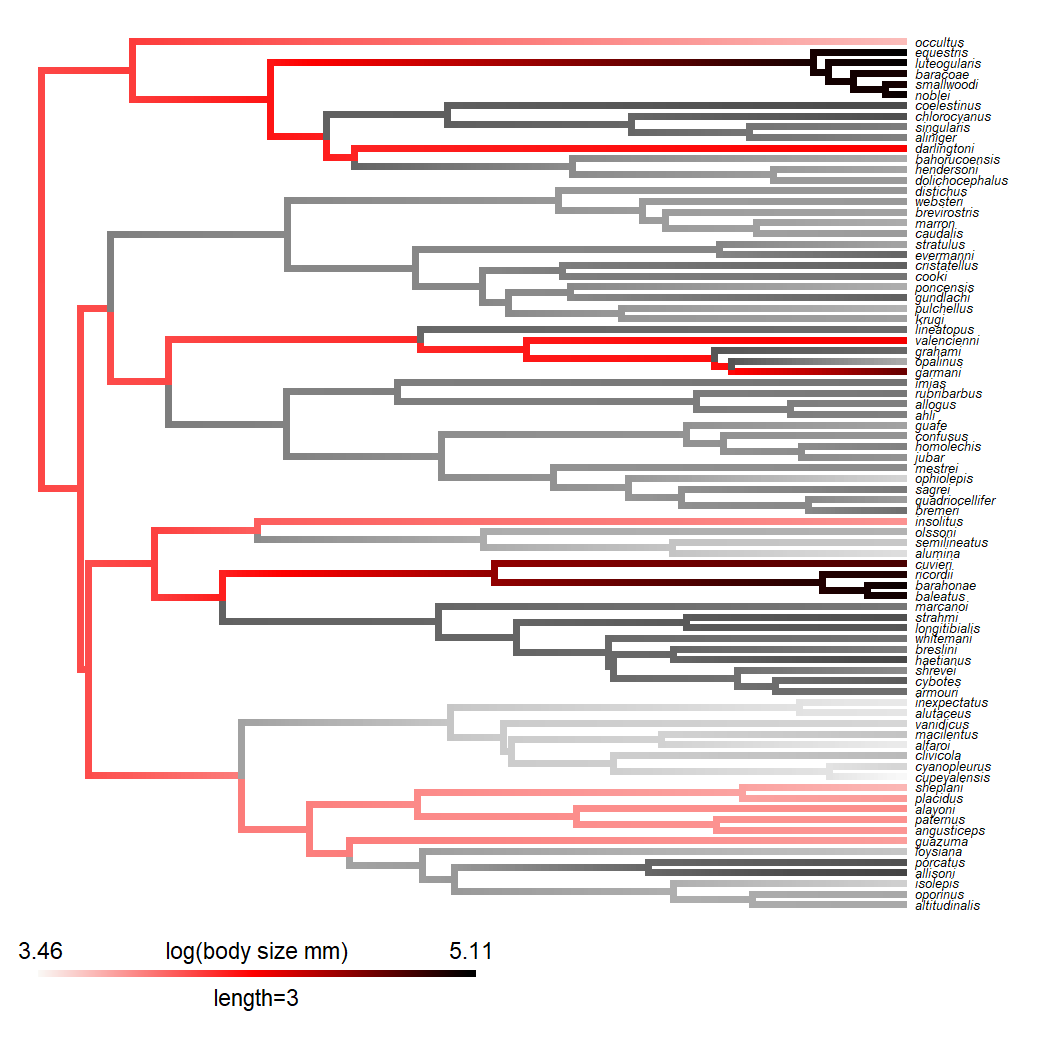## Thursday, June 30, 2022

### Showing certain lineages or clades of a `"contMap"` plot in grey scale

Recently, a phytools user tweeted the following interesting question:

Of course, this is most definitely possible to do in R, alhtough it will involve a bit of knowledge of the structure of the phytools `"contMap"` object in R, as well as a tiny bit of R scripting.

I can see a couple of options for this. The first of this is to duplicate our original `"contMap"` object, recolor the edges of the duplicate `"contMap"` in grey scale, and then combine the two trees.

Let's see how that works.

First, I will load phytools along with an example tree & dataset.

``````library(phytools)
data(mammal.tree)
mammal.tree
``````
``````##
## Phylogenetic tree with 49 tips and 48 internal nodes.
##
## Tip labels:
##   U._maritimus, U._arctos, U._americanus, N._narica, P._lotor, M._mephitis, ...
##
## Rooted; includes branch lengths.
``````
``````data(mammal.data)
``````
``````##               bodyMass homeRange
## U._maritimus     265.0    115.60
## U._arctos        251.3     82.80
## U._americanus     93.4     56.80
## N._narica          4.4      1.05
## P._lotor           7.0      1.14
## M._mephitis        2.5      2.50
``````

Now let's pull out body mass (in kg) and transform it to a log scale.

``````logBodyMass<-setNames(log(mammal.data\$bodyMass),rownames(mammal.data))
``````

Now let's make our first `"contMap"` object showing the evolution of body mass on our phylogenetic tree of mammals.

``````bodyMass.contMap<-contMap(mammal.tree,logBodyMass,plot=FALSE)
bodyMass.contMap<-setMap(bodyMass.contMap,terrain.colors(10))
plot(bodyMass.contMap,fsize=c(0.7,0.9),leg.txt="log(body mass)")
nodelabels(bg="white",frame="circle",cex=0.6)
``````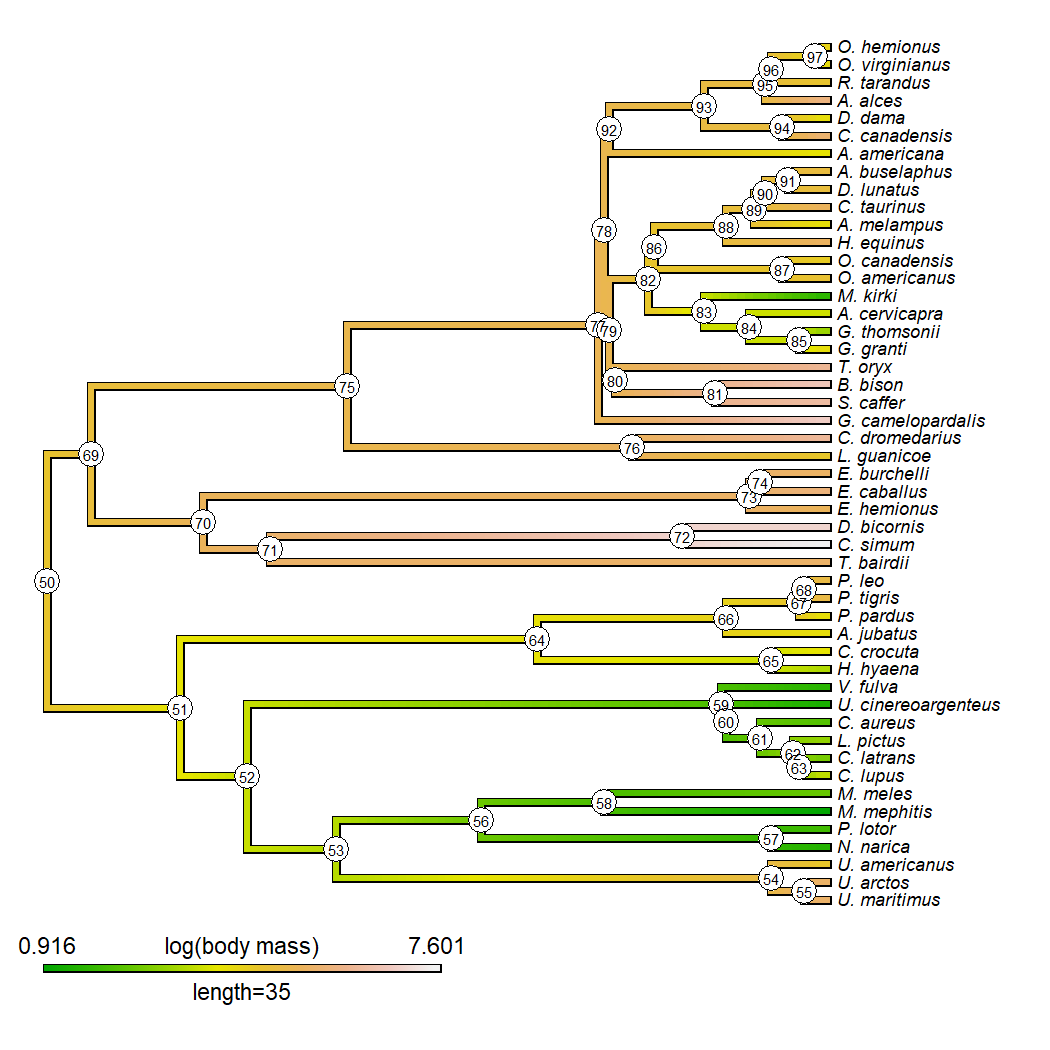Looking at this tree, let's say that (for some reason) we want to recolor to grey scale everything except for Artiodactyla (all descendants of node 75 in our tree), and Canidae (all descendants of node 59). In this example, I'll include the stem edge leading to each of those two clades, but I could've just as easily left it out (or including one but not the other.

As mentioned before, our first step will be to duplicate our `"contMap"` object and convert it to grey scale. To do that, I will use the DescTools function `ColToGrey`, so (obviously) this requires that we have DescTools installed. I could've used `phytools::setMap` and just re-colored the plot, but the idea of `ColToGrey` is to simulate converting our color gradient to grey scale as if we had (for example) printed a color image using a black & white printer, which is (I gather) closer to what the original poster had envisioned.

The other part of this code-chunk involves re-naming the mapped states on our tree. (I decided to just use `"grey0"`, `"grey1"`, … `"grey1000"`.) The purpose of this is to allow us to combine our two `"contMap"` object trees when we are done.

``````grey.contMap<-bodyMass.contMap
grey.contMap\$cols<-setNames(DescTools::ColToGrey(grey.contMap\$cols),
paste("grey",names(grey.contMap\$cols),sep=""))
foo<-function(x){
nn<-names(x)
nn<-paste("grey",nn,sep="")
setNames(x,nn)
}
grey.contMap\$tree\$maps<-lapply(grey.contMap\$tree\$maps,foo)
plot(grey.contMap,fsize=c(0.7,0.9),leg.txt="log(body mass)")
``````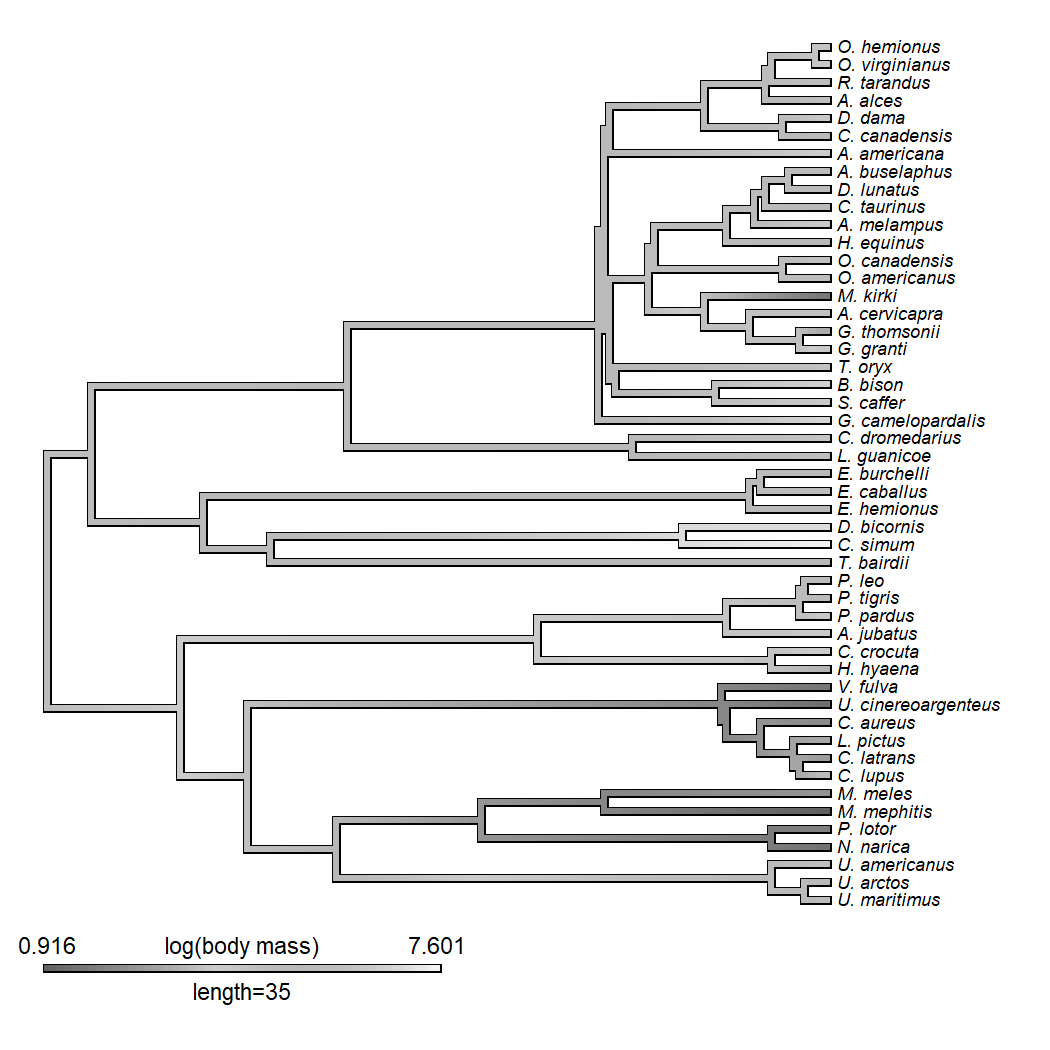``````## we're going to need this later!
ylim<-get("last_plot.phylo",envir=.PlotPhyloEnv)\$y.lim
``````

Now we will identify all edges descended from nodes 75 and 59 (inclusive) as follows.

``````dd<-c(75,getDescendants(mammal.tree,75),59,getDescendants(mammal.tree,59))
``````

Then we can find all other nodes with `setDiff`.

``````ee<-setdiff(getDescendants(mammal.tree,Ntip(mammal.tree)+1),dd)
ee
``````
``````##   51 69 52 64 53 54 56 55  3  1  2 57 58  4  5  6  7 65 66 14 15 16 67 17 68
##  18 19 70 71 73 20 72 21 22 23 74 24 25
``````

Now I'm going to go to create a “merged” `"contMap"` object by substituting the edges ending in the nodes of `ee` with the ones from our object `grey.contMap`.

``````merged<-bodyMass.contMap
for(i in 1:length(ee)){
ii<-which(mammal.tree\$edge[,2]==ee[i])
merged\$tree\$maps[[ii]]<-grey.contMap\$tree\$maps[[ii]]
}
``````

Now I will create an “updated” color gradient for plotting as follows.

``````COLS<-c(merged\$cols,grey.contMap\$cols)
``````

Finally, I'll visualize my `"contMap"`. Here, I'm actually using `plot.simmap` to plot the tree object, and then `add.color.bar` to add on the color legend afterwards.

``````plot(merged\$tree,COLS,split.vertical=TRUE,lwd=6,
outline=FALSE,fsize=0.7,ftype="i",ylim=ylim)
title="log(body mass)",lims=merged\$lims,digits=2,prompt=FALSE,
x=0,y=1-0.08*(Ntip(mammal.tree)-1),fsize=0.9,outline=FALSE,lwd=6)
``````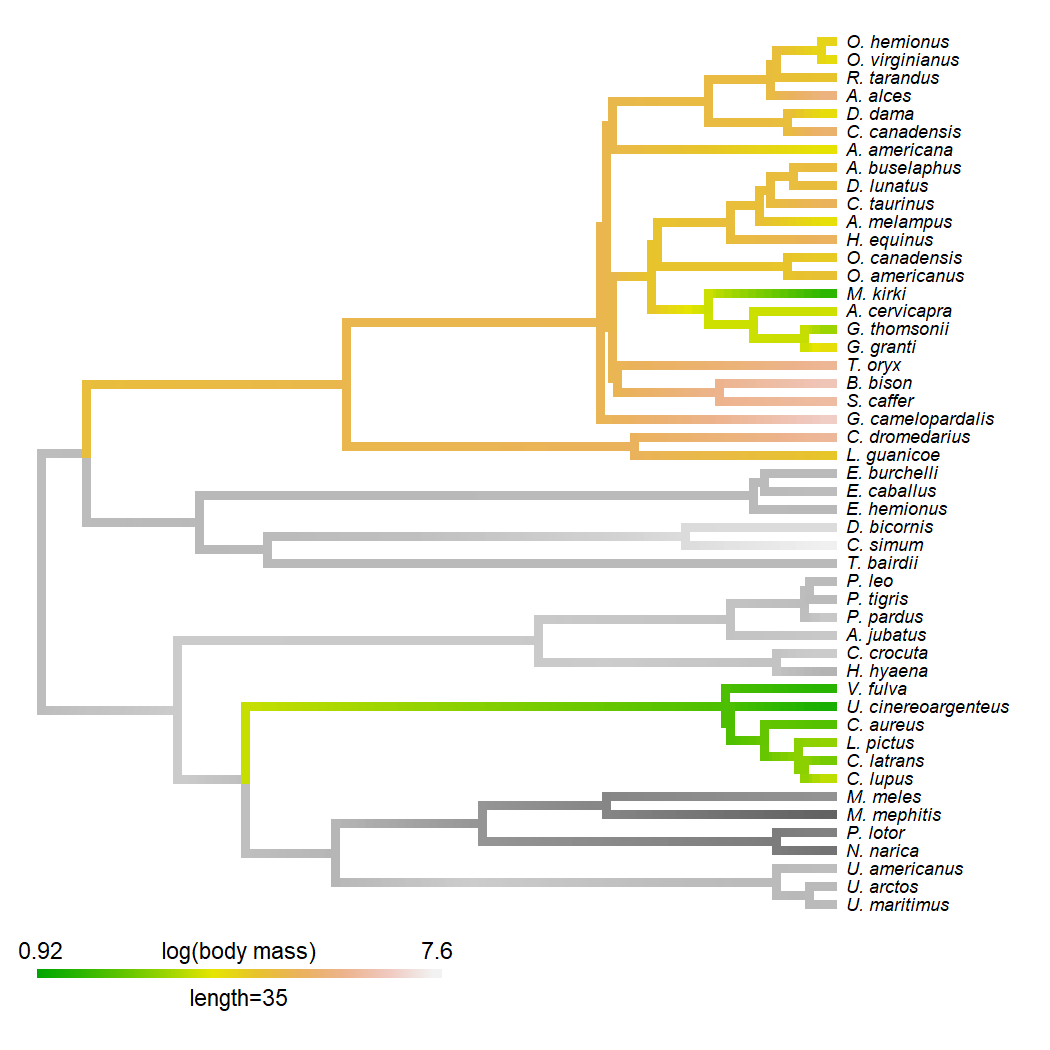That's one way to do it. Here's a totally different one. (I challenge you to figure out how it works!)

``````plot(grey.contMap\$tree,grey.contMap\$cols,
lwd=6,outline=TRUE,fsize=0.7,ftype="i",
ylim=ylim)
transparent<-bodyMass.contMap
for(i in 1:length(ee)) transparent\$tree<-paintBranches(transparent\$tree,
edge=ee[i],state="XX")
COLS<-c("transparent",bodyMass.contMap\$cols)
names(COLS)<-"XX"
xlim<-get("last_plot.phylo",envir=.PlotPhyloEnv)\$x.lim
ftype="off",lwd=6,split.vertical=TRUE)
title="log(body mass)",lims=merged\$lims,digits=2,prompt=FALSE,
x=0,y=1-0.08*(Ntip(mammal.tree)-1),fsize=0.9,lwd=6)
``````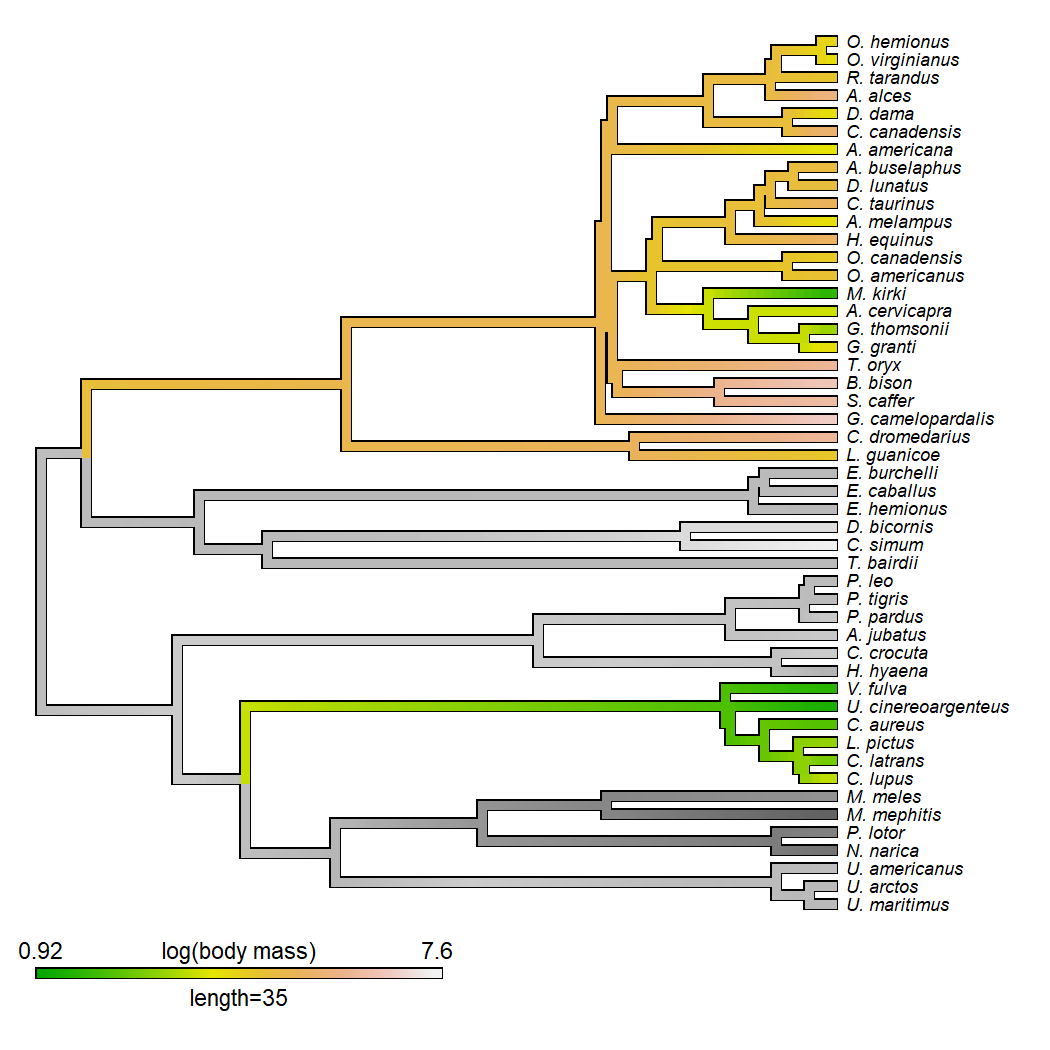In the former example I set `outline=TRUE` and the latter `outline=FALSE`, but either can work either way.

Lastly, here's a fully worked example in which I color all the edges leading to “crown-giant” (the biggest) and “twig” (generally the smallest) anoles in a phylogeny of Caribbean Anolis with the trait log body length.

``````## get data
data(anoletree)
data(anole.data)
## get discrete state
ecomorph<-getStates(anoletree,"tips")
## get ancestors
eco<-names(ecomorph)[which(ecomorph%in%c("Tw","CG"))]
tips<-sapply(eco,function(n,tip.label) which(tip.label==n),
tip.label=unname(anoletree\$tip.label))
nn<-phangorn::Ancestors(anoletree,tips,"all")
nn<-c(unname(tips),sort(unique(unlist(nn))))
ee<-setdiff(getDescendants(anoletree,Ntip(anoletree)+1),nn)
## create initial contMap
anole.contMap<-setMap(contMap(anoletree,
setNames(anole.data\$SVL,rownames(anole.data)),
plot=FALSE,res=200),
c("#FAF9F6","red","black"))
## plot initial contMap
plot(anole.contMap,fsize=c(0.5,0.9),outline=FALSE,
leg.txt="log(body length mm)")
``````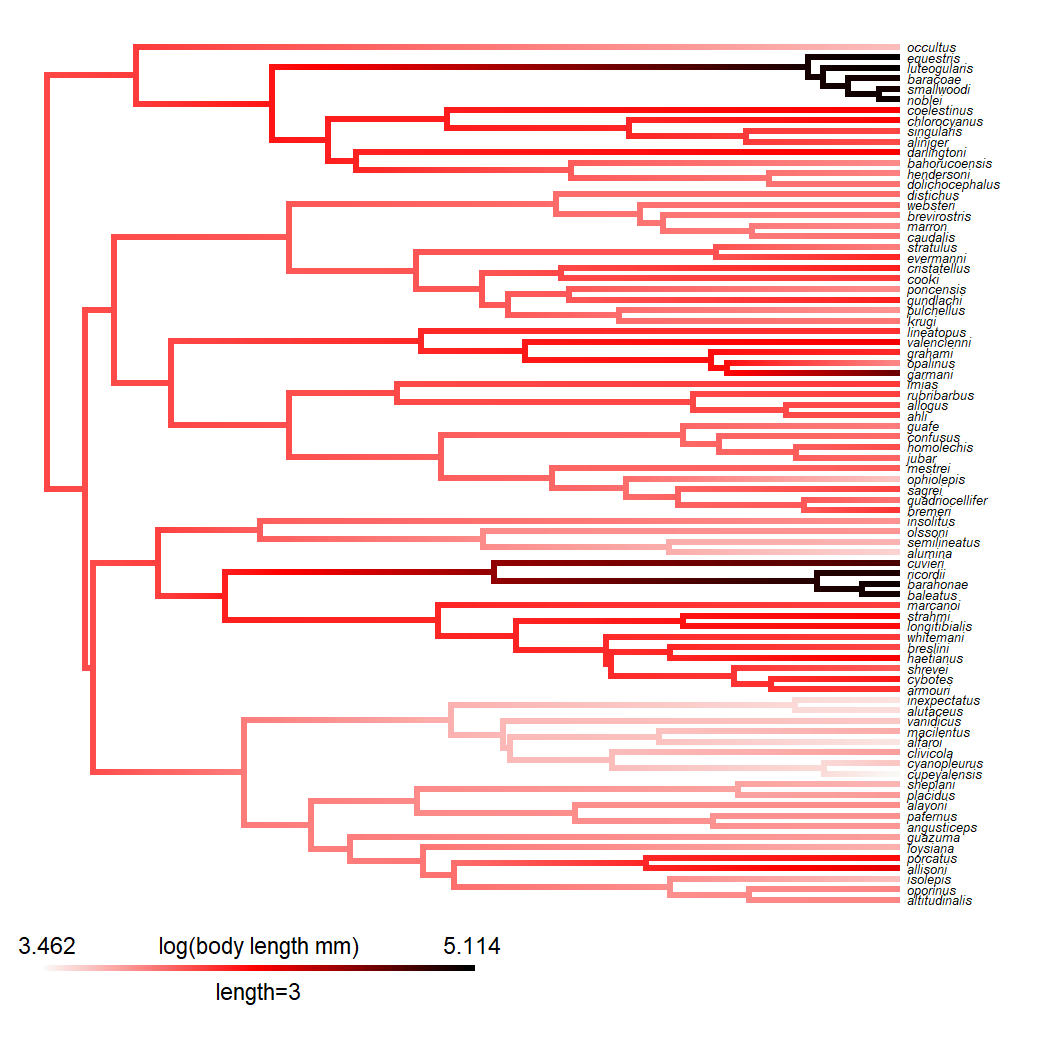``````ylim<-get("last_plot.phylo",envir=.PlotPhyloEnv)\$y.lim
## create grey scale contMap
grey.contMap<-anole.contMap
grey.contMap\$cols<-setNames(DescTools::ColToGrey(grey.contMap\$cols),
paste("grey",names(grey.contMap\$cols),sep=""))
foo<-function(x){
nn<-names(x)
nn<-paste("grey",nn,sep="")
setNames(x,nn)
}
grey.contMap\$tree\$maps<-lapply(grey.contMap\$tree\$maps,foo)
## merge objects
merged<-anole.contMap
for(i in 1:length(ee)){
ii<-which(anoletree\$edge[,2]==ee[i])
merged\$tree\$maps[[ii]]<-grey.contMap\$tree\$maps[[ii]]
}
## merge palette
COLS<-c(merged\$cols,grey.contMap\$cols)
## create plot
plot(merged\$tree,COLS,split.vertical=TRUE,lwd=5,
outline=FALSE,fsize=0.5,ftype="i",ylim=ylim)